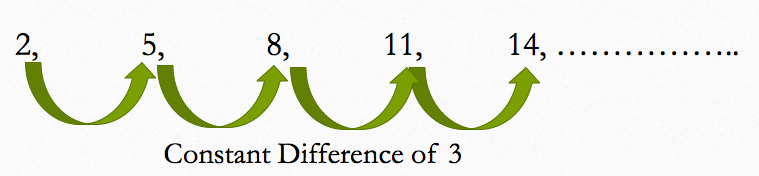## Algebra

#### FUNCTIONS-1

Sequence and Series - 1

Sequence and Series:

In mathematics, sequence is a list of numbers or objects arranged in a particular manner or in a sequential order while series means the sum of the sequence. In this chapter, one by one we will study different types of series which usually appear in competitive exams.

1. Arithmetic Progression:

In an arithmetic progression or popularly known as A.P., the difference of consecutive terms is constant. For e.g. consider the series given below:

2, 5, 8, 11, 14, …….Here the difference between consecutive terms is 3 which is constant.

So, general form of an A.P. is  a, a+d , a+2d, a+3d, …….. a+(n-1)d

Where a is the first term and d is the common term.

So, nth term of an A.P. is given by the formula:Note: if there are three numbers, a,b and c in A.P., then

b – a = c- b  or 2b = a+c

If three numbers are in A.P., then a better approach is to assume the numbers to be a-d, a and a+d

Similarly if four numbers are in A.P., then assume these numbers to a-3d, a-d, a+d and a+3d.

Sum of n terms of an A.P.

Let us say that a given A.P. is of the form :  a, a+d, a+2d, a+3d,……. a+(n-1)d ;

Then the sum of the 1st term and last term = a + a +(n-1)d = 2a +(n-1)d

Sum of the 2nd term and the second last = a+d + a +(n-2)d = 2a + (n-1)d

And so on